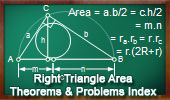# Online Geometry: Area of a right triangle, Theorems and Problems - Table of ContentGeometry Problem 971.
Equilateral Triangle, Rectangle, Common Vertex, Sum of Right Triangles Areas.

Geometry Problem 969.
Equilateral Triangle, Rectangle, Common Vertex, Right Triangles Areas.

Geometry Problem 884: The Pythagorean Curiosity
Right Triangles and squares, fifteen conclusions.

Geometry Problem 813.
Right Triangle Area, Excircles, Cathetus, Tangency Points, Quadrilateral Area.

Geometry Problem 808
Parallelogram, Perpendicular, Right Triangle, Semicircle Area, Diameter.

Geometry Problem 807
Right Triangle Area, Incircle, Tangency Points, Semicircle Area.

Geometry Problem 634
Right Triangle, Altitude, Incenters, Areas.

Geometry Problem 617
Right Triangle Area, Incircle, Incenter, Tangent, 90 Degrees.

Lunes of Hippocrates 1
Area of a right triangle

Lunes of Hippocrates 2
Area of a right triangle

Lunes of Hippocrates 3
Area of a right triangle

Lunes of Hippocrates 4
Area of a right triangle

Problem 540.
Right Triangle, Square, Parallel, Perpendicular, Dissection, Area, Pythagoras.

Problem 539.
Right Triangle, Square, Parallel, Perpendicular, Dissection, Area, Pythagoras.

Problem 534.
Right Triangle, Squares, Perpendicular, Dissection, Areas.

Problem 517.
Right Triangle, Six Squares, Areas.

Lune of Hippocrates Index. Area of a right triangle.

Proposed Problem 327.
Right triangle Area, Incircle, Circumcircle, Square.

Proposed Problem 252.
Rectangle, isosceles right triangle, square, area.

Problem 206. Area of a Right Triangle, Inradius, and Exradius relative to the hypotenuse.

Proposed Problem 205. Right Triangle Area, Exradii relatives to legs or catheti.

Proposed Problem 192. Circle, Diameter, Chord, Perpendicular, Triangle, Area.

De Gua's Theorem
Area of triangles.